Media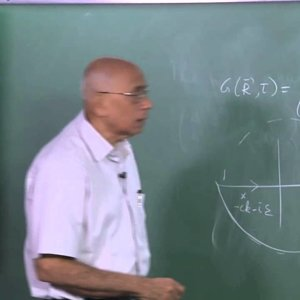Mathematical Physics by Prof. V. Balakrishnan (NPTEL):- Lecture 32: The wave equation (Part I)

• 0
• 0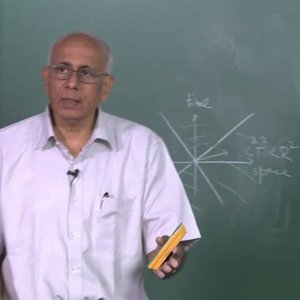Mathematical Physics by Prof. V. Balakrishnan (NPTEL):- Lecture 33: The wave equation (Part II)

• 0
• 0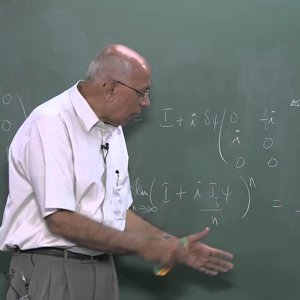Mathematical Physics by Prof. V. Balakrishnan (NPTEL):- Lecture 34: The rotation group and all that (Part I)

• 0
• 0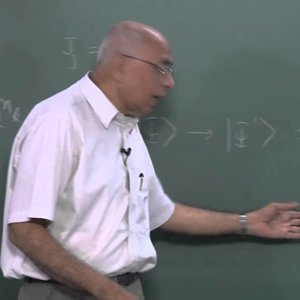Mathematical Physics by Prof. V. Balakrishnan (NPTEL):- Lecture 35: The rotation group and all that (Part II)

• 0
• 0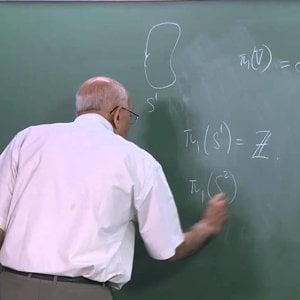Mathematical Physics by Prof. V. Balakrishnan (NPTEL):- Lecture 36: The rotation group and all that (Part III)

• 0
• 0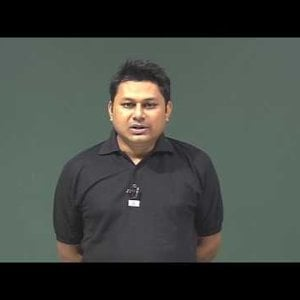Mathematical Physics 1 by Prof. Samudra Roy (NPTEL):- Introduction

• 0
• 0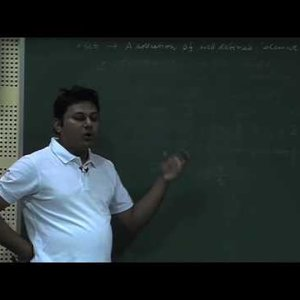Mathematical Physics 1 by Prof. Samudra Roy (NPTEL):- Lecture 1 : Set, Group, Field, Ring

• 0
• 0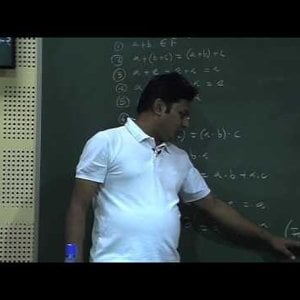Mathematical Physics 1 by Prof. Samudra Roy (NPTEL):- Lecture 2 : Vector Space

• 0
• 0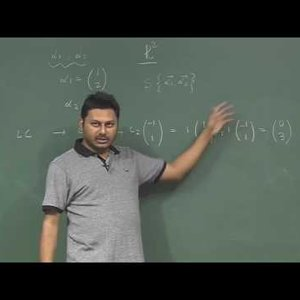Mathematical Physics 1 by Prof. Samudra Roy (NPTEL):- Lecture 3 : Span, Linear combination of vectors

• 0
• 0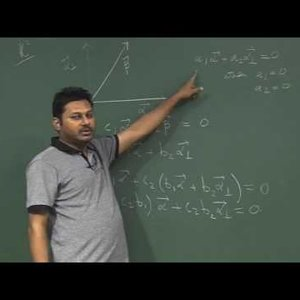Mathematical Physics 1 by Prof. Samudra Roy (NPTEL):- Lecture 4 : Linearly dependent and independent vector, Basis

• 0
• 0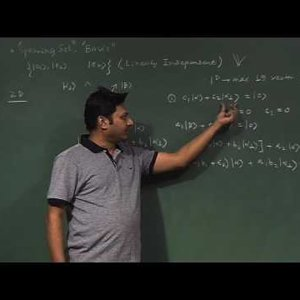Mathematical Physics 1 by Prof. Samudra Roy (NPTEL):- Lecture 5 : Dual Space

• 0
• 0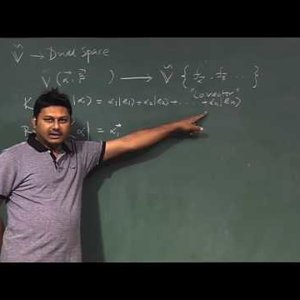Mathematical Physics 1 by Prof. Samudra Roy (NPTEL):- Lecture 6 : Inner Product

• 0
• 0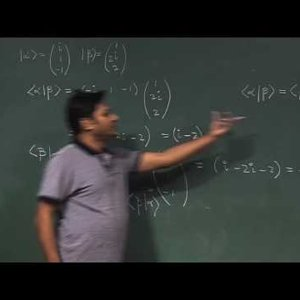Mathematical Physics 1 by Prof. Samudra Roy (NPTEL):- Lecture 7 : Schwarz Inequality

• 0
• 0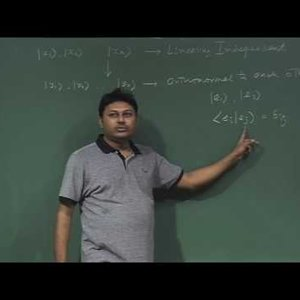Mathematical Physics 1 by Prof. Samudra Roy (NPTEL):- Lecture 8 : Inner product space, Gram- Schmidt Ortho-normalization

• 0
• 0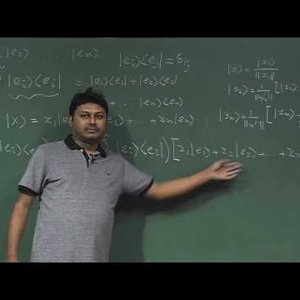Mathematical Physics 1 by Prof. Samudra Roy (NPTEL):- Lecture 9 : Projection operator

• 0
• 0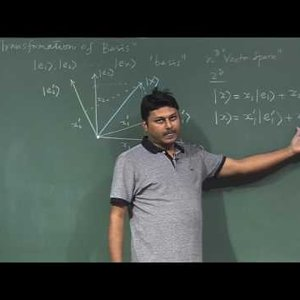Mathematical Physics 1 by Prof. Samudra Roy (NPTEL):- Lecture 10: Transformation of Basis

• 0
• 0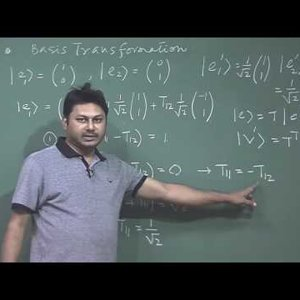Mathematical Physics 1 by Prof. Samudra Roy (NPTEL):- Lecture 11: Transformation of Basis (Continue)

• 0
• 0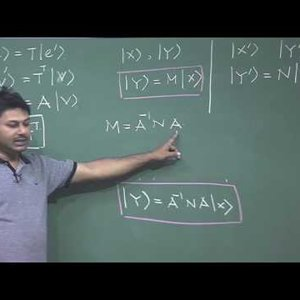Mathematical Physics 1 by Prof. Samudra Roy (NPTEL):- Lecture 12: Unitary transformation, Similarity Transformation

• 0
• 0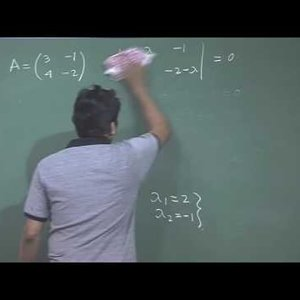Mathematical Physics 1 by Prof. Samudra Roy (NPTEL):- Lecture 13: Eigen Value, Eigen Vectors

• 0
• 0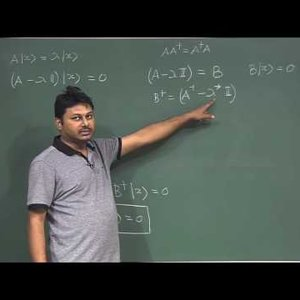Mathematical Physics 1 by Prof. Samudra Roy (NPTEL):- Lecture 14: Normal Matrix

• 0
• 0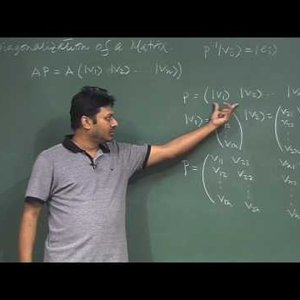Mathematical Physics 1 by Prof. Samudra Roy (NPTEL):- Lecture 15: Diagonalization of a Matrix

• 0
• 0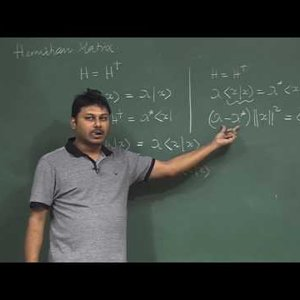Mathematical Physics 1 by Prof. Samudra Roy (NPTEL):- Lecture 16: Hermitian Matrix

• 0
• 0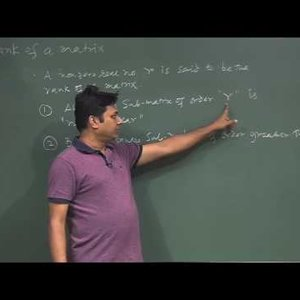Mathematical Physics 1 by Prof. Samudra Roy (NPTEL):- Lecture 17 : Rank of a Matrix

• 0
• 0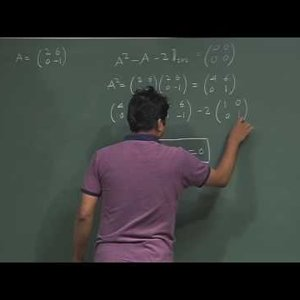Mathematical Physics 1 by Prof. Samudra Roy (NPTEL):- Lecture 18 : Cayley - Hamilton Theorem, Function space

• 0
• 0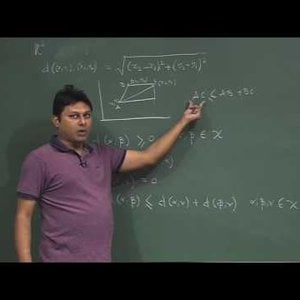Mathematical Physics 1 by Prof. Samudra Roy (NPTEL):- Lecture 19: Metric Space, Linearly dependent –independent functions

• 0
• 0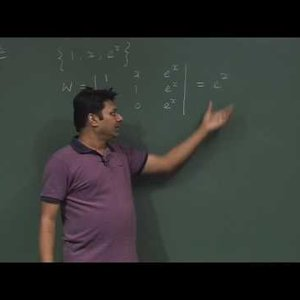Mathematical Physics 1 by Prof. Samudra Roy (NPTEL):- Lecture 20 : Linearly dependent –independent functions (Cont), Inner Product of functions

• 0
• 0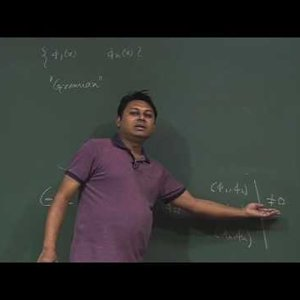Mathematical Physics 1 by Prof. Samudra Roy (NPTEL):- Lecture 21: Orthogonal functions

• 0
• 0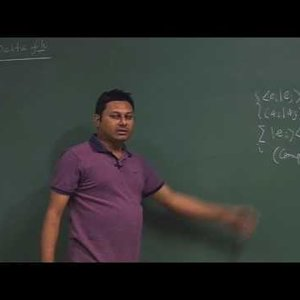Mathematical Physics 1 by Prof. Samudra Roy (NPTEL):- Lecture 22: Delta Function, Completeness

• 0
• 0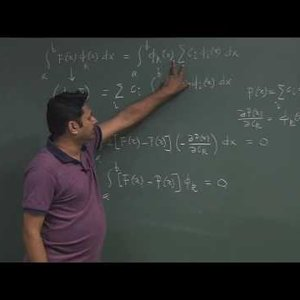Mathematical Physics 1 by Prof. Samudra Roy (NPTEL):- Lecture 23: Fourier Series

• 0
• 0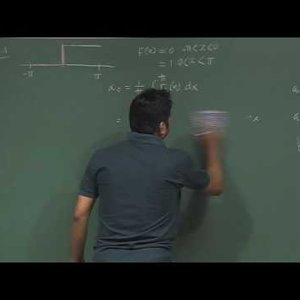Mathematical Physics 1 by Prof. Samudra Roy (NPTEL):- Lecture 24: Fourier Series (Contd.)

• 0
• 0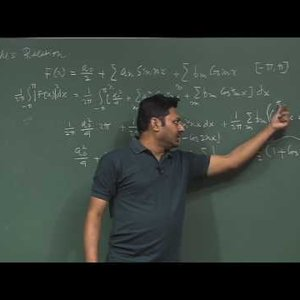Mathematical Physics 1 by Prof. Samudra Roy (NPTEL):- Lecture 25: Parseval Theorem, Fourier Transform

• 0
• 0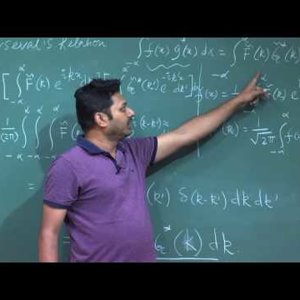Mathematical Physics 1 by Prof. Samudra Roy (NPTEL):- Lecture 26: Parseval Relation, Convolution Theorem

• 0
• 0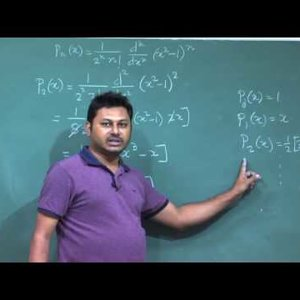Mathematical Physics 1 by Prof. Samudra Roy (NPTEL):- Lecture 27: Polynomial space, Legendre Polynomial

• 0
• 0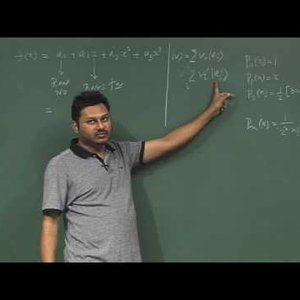Mathematical Physics 1 by Prof. Samudra Roy (NPTEL):- Lecture 28: Monomial Basis, Factorial Basis, Legendre Basis

• 0
• 0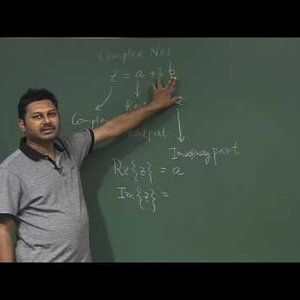Mathematical Physics 1 by Prof. Samudra Roy (NPTEL):- Lecture 29: Complex Numbers

• 0
• 0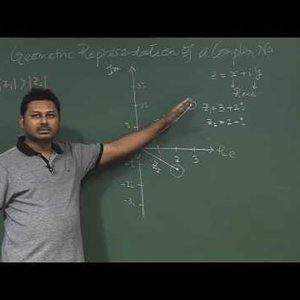Mathematical Physics 1 by Prof. Samudra Roy (NPTEL):- Lecture 30: Geometrical interpretation of complex numbers

• 0
• 0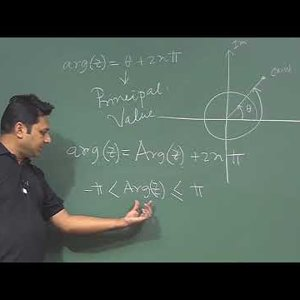Mathematical Physics 1 by Prof. Samudra Roy (NPTEL):- Lecture 31 : de Moivre’s Theorem

• 0
• 0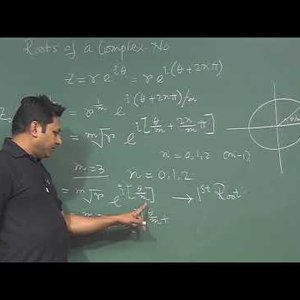Mathematical Physics 1 by Prof. Samudra Roy (NPTEL):- Lecture 32 : Roots of a complex number

• 0
• 0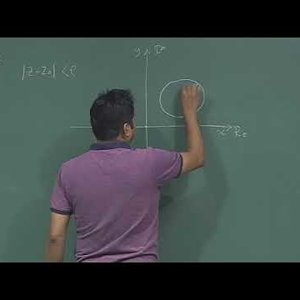Mathematical Physics 1 by Prof. Samudra Roy (NPTEL):- Lecture 33 : Set of complex no, Stereographic projection

• 0
• 0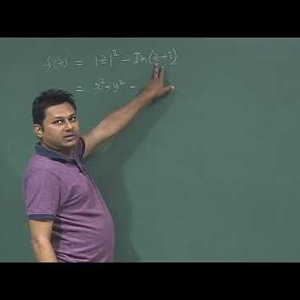Mathematical Physics 1 by Prof. Samudra Roy (NPTEL):-Lecture 34 : Complex Function, Concept of Limit

• 0
• 0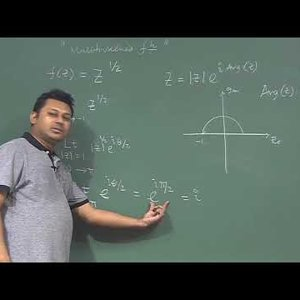Mathematical Physics 1 by Prof. Samudra Roy (NPTEL):- Lecture 35 : Derivative of Complex Function, Cauchy-Riemann Equation

• 0
• 0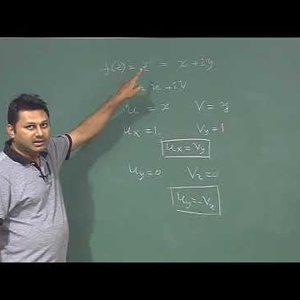Mathematical Physics 1 by Prof. Samudra Roy (NPTEL):- Lecture 36 : Analytic Function

• 0
• 0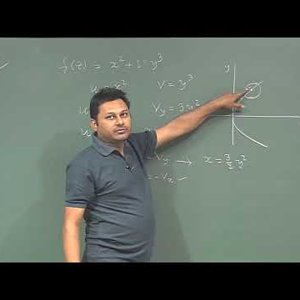Mathematical Physics 1 by Prof. Samudra Roy (NPTEL):- Lecture 37 : Harmonic Conjugate

• 0
• 0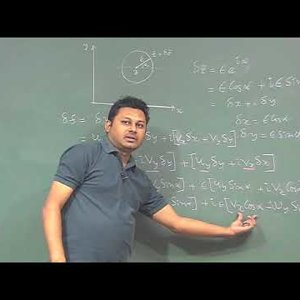Mathematical Physics 1 by Prof. Samudra Roy (NPTEL):- Lecture 38 : Polar form of Cauchy-Riemann Equation

• 0
• 0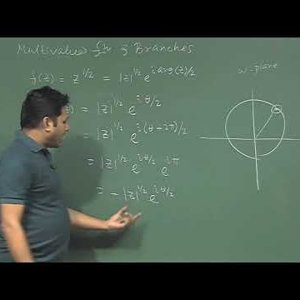Mathematical Physics 1 by Prof. Samudra Roy (NPTEL):- Lecture 39 : Multi-valued function and Branches

• 0
• 0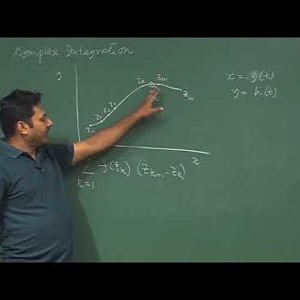Mathematical Physics 1 by Prof. Samudra Roy (NPTEL):- Lecture 40 : Complex Line Integration, Contour, Regions

• 0
• 0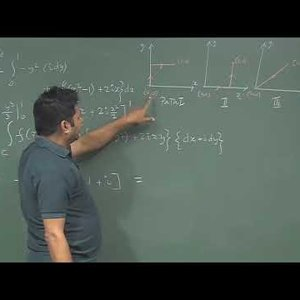Mathematical Physics 1 by Prof. Samudra Roy (NPTEL):- Lecture 41: Complex Line Integration(Cont.)

• 0
• 0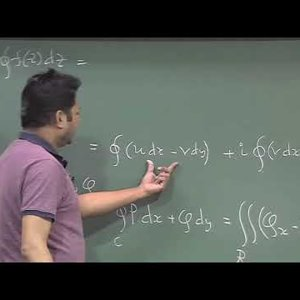Mathematical Physics 1 by Prof. Samudra Roy (NPTEL):-Lecture 42: Cauchy-Goursat Theorem

• 0
• 0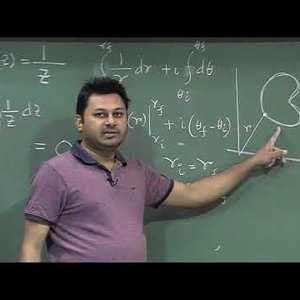Mathematical Physics 1 by Prof. Samudra Roy (NPTEL):- Lecture 43: Application of Cauchy-Goursat Theorem

• 0
• 0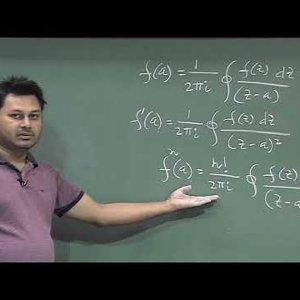Mathematical Physics 1 by Prof. Samudra Roy (NPTEL):- Lecture 44: Cauchy’s Integral Formula

• 0
• 0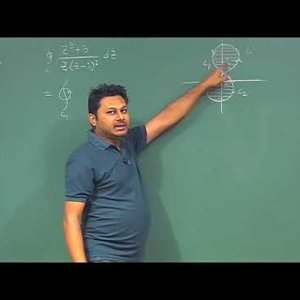Mathematical Physics 1 by Prof. Samudra Roy (NPTEL):- Lecture 45: Cauchy’s Integral Formula (Contd.)

• 0
• 0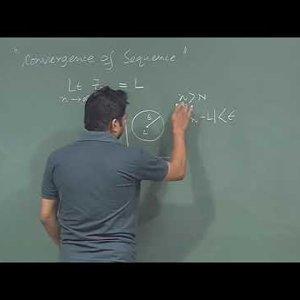Mathematical Physics 1 by Prof. Samudra Roy (NPTEL):- Lecture 46: Series and Sequence

• 0
• 0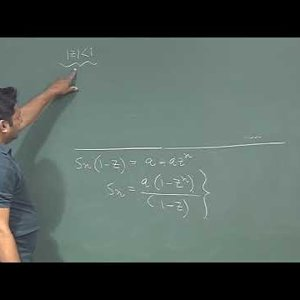Mathematical Physics 1 by Prof. Samudra Roy (NPTEL):- Lecture 47: Series and Sequence (Contd.)

• 0
• 0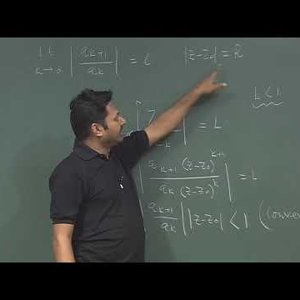Mathematical Physics 1 by Prof. Samudra Roy (NPTEL):- Lecture 48:Circle and radius of convergence

• 0
• 0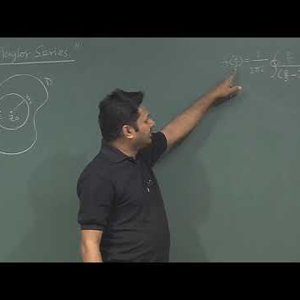Mathematical Physics 1 by Prof. Samudra Roy (NPTEL):- Lecture 49: Taylor Series

• 0
• 0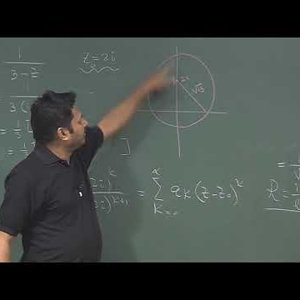Mathematical Physics 1 by Prof. Samudra Roy (NPTEL):- Lecture 50: Classification of singularity

• 0
• 0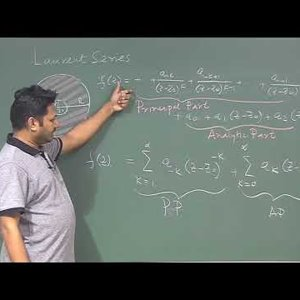Mathematical Physics 1 by Prof. Samudra Roy (NPTEL):- Lecture 51: Laurent Series, Singularity

• 0
• 0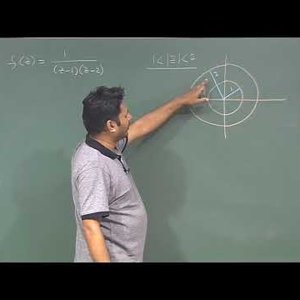Mathematical Physics 1 by Prof. Samudra Roy (NPTEL):- Lecture 52: Laurent series expansion

• 0
• 0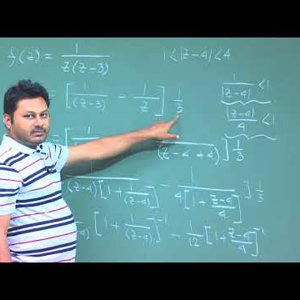Mathematical Physics 1 by Prof. Samudra Roy (NPTEL):- Lecture 53: Laurent series expansion (Cont), Concept of Residue

• 0
• 0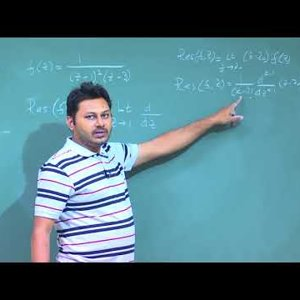Mathematical Physics 1 by Prof. Samudra Roy (NPTEL):- Lecture 54: Classification of Residue

• 0
• 0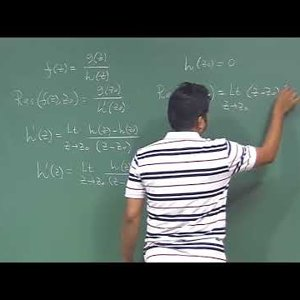Mathematical Physics 1 by Prof. Samudra Roy (NPTEL):- Lecture 55: Calculation of Residue for quotient

• 0
• 0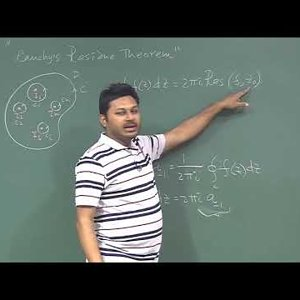Mathematical Physics 1 by Prof. Samudra Roy (NPTEL):- Lecture 56 : Cauchy’s Residue Theorem

• 0
• 0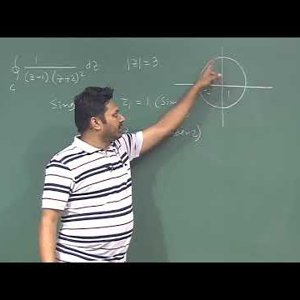Mathematical Physics 1 by Prof. Samudra Roy (NPTEL):-Lecture 57 : Cauchy’s Residue Theorem (Cont)

• 0
• 0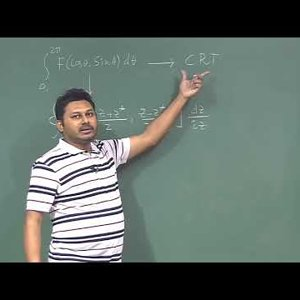Mathematical Physics 1 by Prof. Samudra Roy (NPTEL):- Lecture 58 : Real Integration using Cauchy’s Residue Theorem

• 0
• 0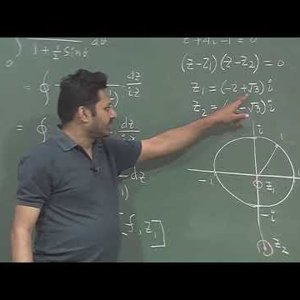Mathematical Physics 1 by Prof. Samudra Roy (NPTEL):- Lecture 58 : Real Integration using Cauchy’s Residue Theorem (Contd. 1)

• 0
• 0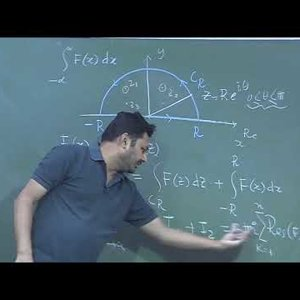Mathematical Physics 1 by Prof. Samudra Roy (NPTEL):- Lecture 58 : Real Integration using Cauchy’s Residue Theorem (Contd. 2)

• 0
• 0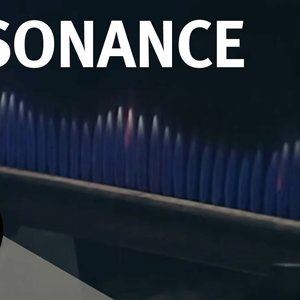Visualising Resonance - Christmas Lectures with Leonard Maunder

• 0
• 0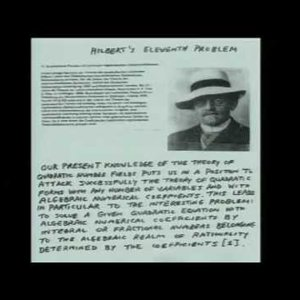The Ramanujan Conjecture and some diophantine equations - Peter Sarnak

• 0
• 0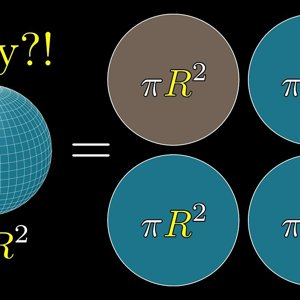But WHY is a sphere's surface area four times its shadow? - YouTube

• 0
• 1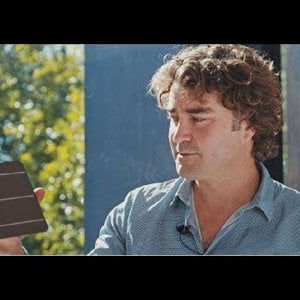The Path to Perovskite on Silicon PV | Prof. Henry Snaith

• 0
• 0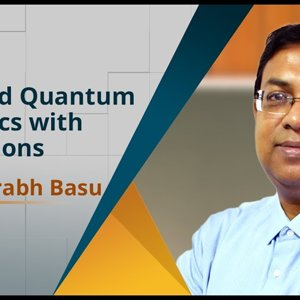Advanced Quantum Mechanics with Applications by Prof. Saurabh Basu (NPTEL):- Introduction Video

• 0
• 0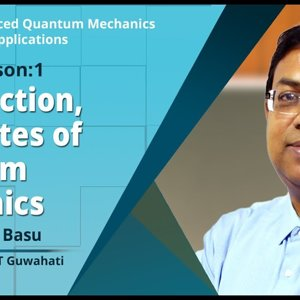Advanced Quantum Mechanics with Applications by Prof. Saurabh Basu (NPTEL):- Introduction , Postulates of Quantum Mechanics

• 0
• 0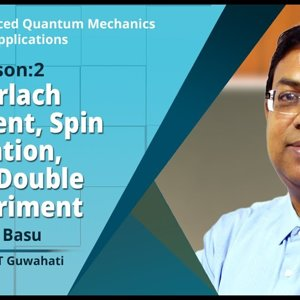Advanced Quantum Mechanics with Applications by Prof. Saurabh Basu (NPTEL) Stern Gerlach Experiment, Spin Quantization, Young's Double Slit Experiment

• 0
• 0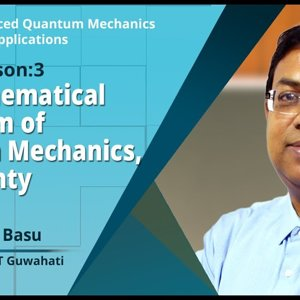Advanced Quantum Mechanics with Applications by Prof. Saurabh Basu (NPTEL):- The Mathematical Formalism of Quantum Mechanics, Uncertainty Principle

• 0
• 0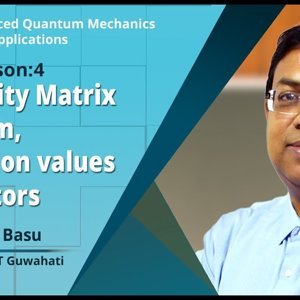Advanced Quantum Mechanics with Applications by Prof. Saurabh Basu (NPTEL):- The Density Matrix Formalism, Expectation values of Operators

• 0
• 0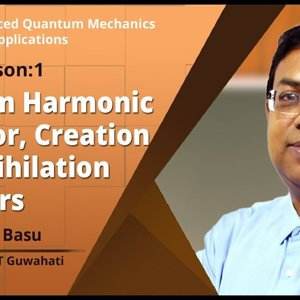Advanced Quantum Mechanics with Applications by Prof. Saurabh Basu (NPTEL):- Qunatum Harmonic Oscillator, Creation and annihilation Operators

• 0
• 0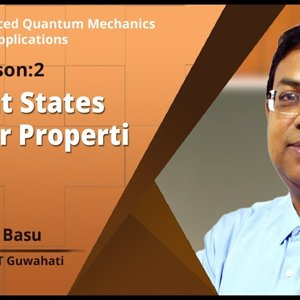Advanced Quantum Mechanics with Applications by Prof. Saurabh Basu (NPTEL):- Coherent States and their Properties

• 0
• 0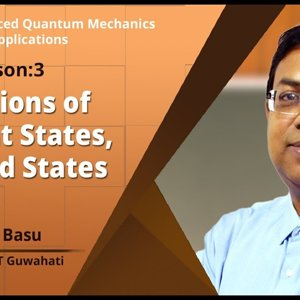Advanced Quantum Mechanics with Applications by Prof. Saurabh Basu (NPTEL):- Applications of Coherent States, squeezed states

• 0
• 0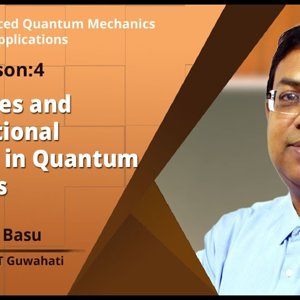Advanced Quantum Mechanics with Applications by Prof. Saurabh Basu (NPTEL):- Symmetries and Conservational Principles in Quantum Mechanics

• 0
• 0Advanced Quantum Mechanics with Applications by Prof. Saurabh Basu (NPTEL):- Rotation Operator and Invariance of Angular Momentum, Parity

• 0
• 0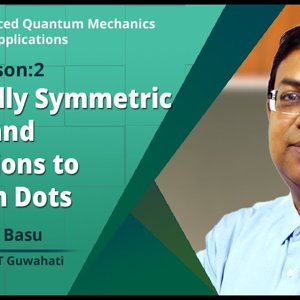Advanced Quantum Mechanics with Applications by Prof. Saurabh Basu (NPTEL):- Spherically Symmetric System and Applications to quantum dots

• 0
• 0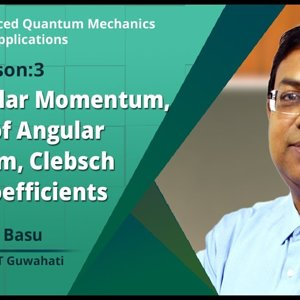Advanced Quantum Mechanics with Applications by Prof. Saurabh Basu :- Spin Angular Momentum, Addition of Angular Momentum, Clebsch gordan coefficients

• 0
• 0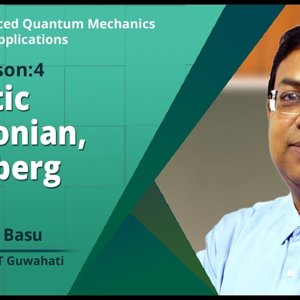Advanced Quantum Mechanics with Applications by Prof. Saurabh Basu (NPTEL):- Magnetic Hamiltonian, Heisenberg Model

• 0
• 0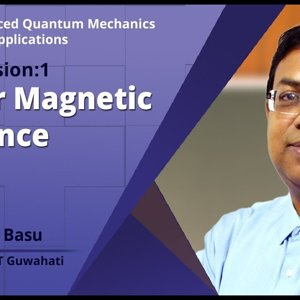Advanced Quantum Mechanics with Applications by Prof. Saurabh Basu (NPTEL):- Nuclear Magnetic Resonance (NMR)

• 0
• 0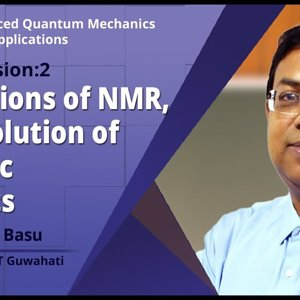Advanced Quantum Mechanics with Applications by Prof. Saurabh Basu (NPTEL):- Applications of NMR, time evolution of Magnetic Moments

• 0
• 0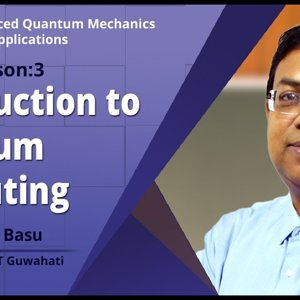Advanced Quantum Mechanics with Applications by Prof. Saurabh Basu (NPTEL):- Introduction to Quantum Computing

• 0
• 0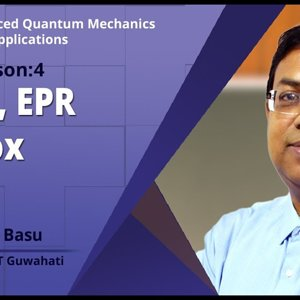Advanced Quantum Mechanics with Applications by Prof. Saurabh Basu (NPTEL):- Qubits, EPR Paradox

• 0
• 0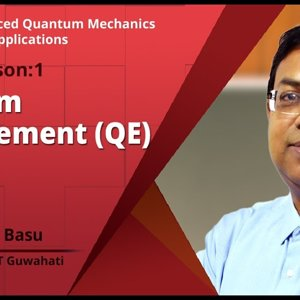Advanced Quantum Mechanics with Applications by Prof. Saurabh Basu (NPTEL):- Quantum Entanglement (QE)

• 0
• 0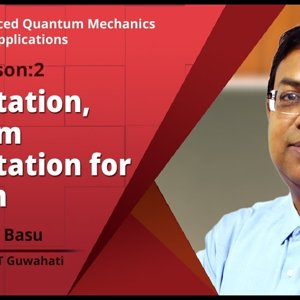Advanced Quantum Mechanics with Applications by Prof. Saurabh Basu (NPTEL):- Teleportation, Quantum Teleportation for one spin

• 0
• 0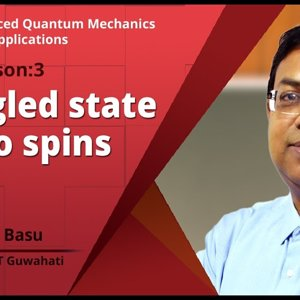Advanced Quantum Mechanics with Applications by Prof. Saurabh Basu (NPTEL):- Entangled state for two spins

• 0
• 0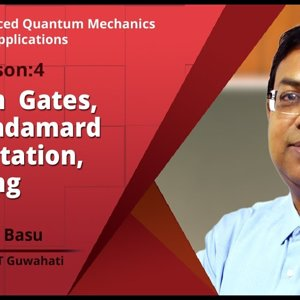Advanced Quantum Mechanics with Applications by Prof. Saurabh Basu (NPTEL):- Quantum Gates, Walsh Hadamard Transportation, No cloning theorem

• 0
• 0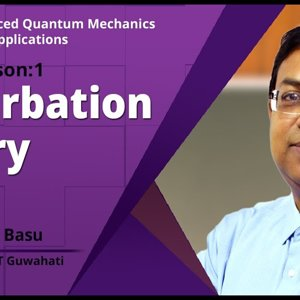Advanced Quantum Mechanics with Applications by Prof. Saurabh Basu (NPTEL):- Perturbation Theory

• 0
• 0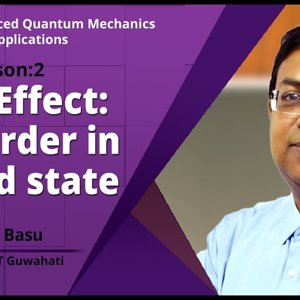Advanced Quantum Mechanics with Applications by Prof. Saurabh Basu (NPTEL):- Stark Effect: First order in ground state

• 0
• 0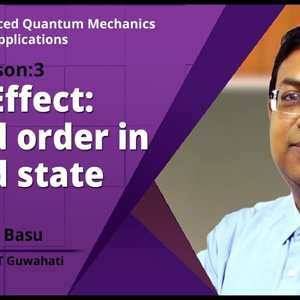Advanced Quantum Mechanics with Applications by Prof. Saurabh Basu (NPTEL):- Stark Effect: Second order in ground state

• 0
• 0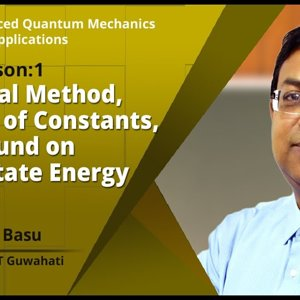• 0
• 0